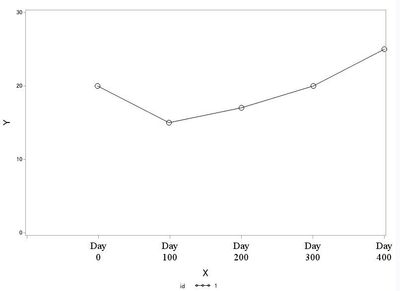## How Can I Delete Un-Needed Blank on Axis X in PROC GPLOT?

Hi, there:

I have trouble to adjust axis X's attribute.

``````data test;
input id x y @@;
datalines;
1 -1 20 1 99 15 1 200 17 1 301 20 1 400 25
;
run;
symbol1 color=black value=circle repeat=1 interpol=join height=2;
axis1 minor=none label=(angle=90 rotate=0 height=2cells "Y")
order=0 to 30 by 10;
axis99 minor=none label=(angle=0 rotate=0 height=2cells "X")
order=-100 to 400 by 100
value=(angle=0 rotate=0 height=2cells font="Times New Roman"
tick=1 height=2cells " " justify=center height=2cells " "
tick=2 height=2cells "Day" justify=center height=2cells "0"
tick=3 height=2cells "Day" justify=center height=2cells "100"
tick=4 height=2cells "Day" justify=center height=2cells "200"
tick=5 height=2cells "Day" justify=center height=2cells "300"
tick=6 height=2cells "Day" justify=center height=2cells "400"
);
proc gplot data=test;
plot y*x=id / haxis=axis99 vaxis=axis1;
run;
quit;``````On axis X, I want these ticks (Day0, Day100, Day200, Dya300, Day400), but I don't need such as blank on Day0's left (I want smaller one).

In my dataset, there is X=-1 (lesser than Day0), so in this case, using OFFSETMIN= in PROC SGPLOT acts well, I know.

2 REPLIES 2

## Re: How Can I Delete Un-Needed Blank on Axis X in PROC GPLOT?

Since your X variable is continuous* rather than nominal, it might be acceptable to display intermediate tick marks.

In this case you could change the ORDER= option of AXIS99 to, say,

``order=-10 to 400 by 10``

and hide the repetitive part of the VALUE= specification in a macro:

``````    value=(angle=0 rotate=0 height=2cells font="Times New Roman"
%ticks
);``````

Macro definition (depending on the ORDER= specification, of course):

``````%macro ticks;
%local t;
%do t=1 %to 42;
%if %sysfunc(mod(&t,10))=2 %then
tick=&t height=2cells "Day" justify=center height=2cells "%eval(-20+&t*10)";
%else
tick=&t height=2cells " " justify=center height=2cells " ";
%end;
%mend ticks;``````

Other suggestions (some of which allow the omission of the intermediate tick marks) can be found in this paper.

*(EDIT: in the sense that its values can slightly depart from the few tick mark values you want to display)

## Re: How Can I Delete Un-Needed Blank on Axis X in PROC GPLOT?

Hi, FreelanceReinhard:

Thanks so much.

In order to delete intermediate ticks, I try yours.

Please wait until I report this result.

Thanks again.

Discussion stats
• 2 replies
• 661 views
• 1 like
• 2 in conversation